[BACK]Sound & VibrationDOI: 10.32604/sv.2021.011387

ARTICLE

Civil Engineering Department, Motilal Nehru National Institute of Technology Allahabad, Prayagraj, 211004, India
*Corresponding author: Ravi Joshi. Email: manas1631@gmail.com
Received: 06 May 2020; Accepted: 13 August 2020

Abstract: Ply-by-ply failure analysis of symmetric and anti-symmetric laminates under uniform sinusoidal transverse dynamic loading is performed for a specified duration. The study investigates the first ply failure load, followed by the detection of successive ply failures along with their failure modes using various failure theories. Some of the well-established failure theories, mostly used by the researchers, are considered for the failure prediction in laminates. The finite element computational model based on higher order shear deformation displacement field is used for the failure analysis and the complete methodology is computer coded using FORTRAN. The ply-discount stiffness reduction scheme is employed to modify the material properties of the failed lamina. The failure theories used in the analysis are compared according to their ability to predict failure load, failed ply, failure mode and progression of failure. The failure analysis is performed for both the cross-ply and angle-ply laminates with all edges simply supported and clamped. The significance of fibre orientation and stacking sequence in terms of the strength of a laminate and failure progression is also highlighted.

Keywords: Composite laminates; failure mode; dynamic load; finite element analysis (FEA)

1  Introduction

The failure analysis of laminated composite plates under dynamic load is essential as the laminated plates are used as a primary load-bearing member in aircraft, marine, civil and aerospace structures. The applications of composite structures have now extended to the traditional automotive industry also. The increasing applications of composite structures demand a reliable performance assessment of these structures. Failure of a composite material due to its complexities has always been a subject of research ever since its inception. A fibrous composite laminate fails progressively, starting from micro-level failure such as fibre failure, matrix failure, fibre matrix pull-out, etc. to laminate level failure in the form of cracks in the constituent lamina.

The present study investigates the ply-by-ply failure analysis of laminates under dynamic load based on higher order shear deformation theory (HSDT) using the finite element method. The study aims to analyse the failure of laminates under dynamic load for a specified duration. For all the laminates investigated, the load is applied for a duration of 0.4 sec and checked for first ply failure. The first ply failure loads of laminates are calculated for the given load duration and the progress of failure is observed after the first ply failure load has been removed. The dynamic response of the laminates is obtained using the Newmark-beta direct integration scheme.

2  Failure Theories

The failure theories used for the failure prediction of composite laminates are classified in three criteria viz. independent, interactive and mode determining failure criteria 

2.1 Independent Failure Criteria

This is the oldest failure criteria used for composite laminates. This failure criteria assume failure if any of the stress or strain component reaches its maximum permissible value. The maximum stress criterion is an example of this category.

2.1.1 Maximum Stress Theory

This failure criterion assumes failure when any of the six stress components reaches its ultimate allowable value while the other stress components may be well within their limit. The safe conditions mathematically according to the maximum stress theory can be written as:

XC<σ11<XT (1)

YC<σ22<YT (2)

ZC<σ33<ZT (3)

|τ23|<Q (4)

|τ31|<R (5)

|τ12|<S (6)

where σ11,σ22,σ33,τ23,τ31andτ12 are the stress components in the material coordinate axis. X, Y and Z are the maximum permissible values of stresses in the material coordinate axis with subscript T and C denoting tension and compression. Q, R and S are the maximum permissible values of shear stresses.

2.2 Interactive Failure Criteria

In this criteria, a single polynomial usually quadratic equation with all the stress components is used to predict the lamina’s failure. This failure criteria better correlate the theoretical values and the experiments by including all the stress components. Tsai-Hill, Azzi-Tsai, Hoffman and Tsai-Wu failure theories fall in this category.

2.2.1 Tsai-Hill Theory

This criterion is an extension of Von-Mises distortional energy failure criterion applied to the anisotropic material. The theory suggests failure if the following equation is satisfied.

f(σij)=F( σ22σ33)2+G( σ33σ11)2+H( σ11σ22)2+2Lτ232+2Mτ132+2Nτ122=1(7)

where F, G, H, L, M and N are the material strength parameters that are determined from a series of thought experiments.

The reduced form of failure criterion for plane stress condition can be represented as follows:

(σ11X)2+(σ22Y)2+(σ11X)(σ22X)+(τ12S)2=1 (8)

where X, Y and S are ultimate in-plane stresses.

2.2.2 Azzi-Tsai theory

This failure theory is the same as Tsai-Hill theory, but the absolute value of the two normal stresses product is taken; hence the failure criterion can be written as:

(σ11X)2+(σ22Y)2+(1X)2|σ1122|+(τ12S)2=1 (9)

2.2.3 Hoffman Theory

The Hoffman failure theory’s strength parameters include odd functions of normal stresses, unlike the Tsai-Hill expression. Therefore the failure condition for plane stress is given by:

(1XT1XC)σ11+(1YT1YC)σ111XTXCσ11σ22

+1XTXC(σ11)2+1YTYC(σ22)2+1S2(τ12)2=1 (10)

2.2.4 Tsai-Wu Theory

This failure criterion consists of the scalar polynomial of stress tensors and strength. The following expression gives the failure surface:

f(σi) =Fiσi+Fijσij=1 (11)

The following expression gives the failure surface expression for plane stress condition:

(1XT1XC)σ11+(1YT1YC)σ11(1XTXC×1YTYC)σ11σ22+1XTXC(σ11)2+1YTYC(σ22)2

+1S2(τ12)2=1 (12)

2.3 Mode Determining Failure Criteria

The independent and interactive failure criteria do not provide any information about the failure mode. The mode determining failure criteria predict the failure along with the failure mode. These failure criteria include separate polynomial equations for each failure mode viz. fibre tension, matrix tension, fibre compression, matrix compression, etc. Hashin-Rotem, Rotem and Hashin failure criteria are some of the examples of mode determining failure criteria.

2.3.1 Hashin-Rotem Failure Criterion

Hashin-Rotem criterion assumes failure in a lamina if any of these equations for their corresponding modes of failure satisfies.

Fibre failure (tension), i.e., σ11 ≥ 0

σ11XT=1 (13)

Fibre failure (compression), i.e., σ11 < 0

σ11XC=1 (14)

Matrix failure (tension), i.e., σ22 > 0

(σ22YT)2+(τ12S)2=1 (15)

Matrix failure (compression), i.e., σ22 < 0

(σ22YC)2+(τ12S)2=1 (16)

2.3.2 Rotem Failure Criterion

In Rotem’s failure criterion, the lamina's axial matrix properties are also considered for the matrix mode failure. The failure criterion is given by:

Fibre failure (tension), i.e., σ11 ≥ 0

σ11XT=1 (17)

Fibre failure (compression), i.e., σ11 < 0

σ11XC=1 (18)

Matrix failure (tension), i.e., σ22 > 0

(Emε11ε11YT)2+(σ22YT)2+(τ12S)2=1 (19)

Matrix failure (compression), i.e., σ22 < 0

(Emε11ε11YC)2+(σ22YC)2+(τ12S)2=1 (20)

where Em is the young’s modulus of matrix.

2.3.3 Hashin Failure Criterion

In Hashin failure theory, the linear terms in the expression for fibre failure modes of Hashin-Rotem failure theory are replaced by quadratic terms and a strength parameter of a different plane is considered for matrix compression failure mode. The expressions for Hashin failure criterion are given as:

Fibre failure (tension), i.e., σ11 ≥ 0

(σ11XT)2+(τ12S)2=1 (21)

Fibre failure (compression), i.e., σ11 < 0

(σ11XC)2=1 (22)

Matrix failure (tension), i.e., σ22 > 0

(σ22YT)2+(τ12S)2=1 (23)

Matrix failure (compression), i.e., σ22 < 0

((YC2Q)21)(σ22YC)+(τ12S)2+(σ222Q)2=1 (24)

3  Failure Modes

For failure criteria other than the mode determining, failure indices are to be determined to predict the failure mode. The failure indices are calculated as follows:

H1=F1F11+F11(σ11)2 (fibre failure); and H2=F2F22+F22(σ22)2 (matrix failure) (25)

The largest among H1 and H2 is considered as the dominant failure mode and the respective elastic constants are reduced to zero. The failure indices parameters for different failure criteria are given as follows:

3.1 Maximum Stress Criterion

F1=1XT1XC,F2=1YT1YC,F12=F1F22,F11=1XTXC,F22=1YTYC (26)

3.2 Hoffman Criterion

F1=1XT1XC,F2=1YT1YC,F12=12XTXC,F11=1XTXC,F22=1YTYC (27)

3.3 Tsai-Hill Criterion & Azzi-Tsai Criterion

F1=0,F2=0,F12=12X2,F11=1X2,F22=1Y2 (28)

3.4 Tsai-Wu Criterion

F1=1XT1XC,F2=1YT1YC,F12=F11F222,F11=1XTXC,F22=1YTYC (29)

4  Mathematical Formulation

The displacement field, according to HSDT of a point in an anisotropic laminated composite plate is expressed as :

{U(x,y,z)=u+zθx+z2θx+z3φxV(x,y,z)=v+zθy+z2θy+z3φyW(x,y,z)=w(x,y) (30)

U, V and W represent displacements at any point in x, y, z directions respectively. The mid-plane translation component in the x, y and z axis are denoted by u, v & w, respectively. θx and θydenotes the rotational component of normal to mid-plane about y and x axis, respectively. θx and θy are the higher order translation functions, and φx and φy are the higher-order rotation function. The transverse shear stress free surfaces condition is satisfied by the above displacement field. For a laminated plate comprising layers of orthotropic plies or an orthotropic plate, the transverse shear stress-free surfaces condition requires the corresponding shear strains to be zero at the surfaces, i.e.,

γyz(x,y,±h2)=0andγxz(x,y,±h2)=0 (31)

γyz=Vz+Wyandγxz=Uz+Wx (32)

Equating Eqs. (31) and (32) and substituting for U, V, W from Eq. (30) we obtain θx and θy = 0 and the displacement field reduces to

{U(x,y,z)=u+zθx+z3φxV(x,y,z)=v+zθy+z3φyW(x,y,z)=w(x,y) (33)

Eq. (33) is the reduced seven degrees of freedom displacement field formulated from the original nine degrees of freedom displacement field (Eq (30)) is used in the present investigation. The finite element computational model and stress-strain constitution model is well known and can be referred from the literature . The equation of motion for undamped forced vibration is given by

[M]{d¨}+[K]{d}={P} (34)

The undamped free vibration equation is given by

[M]{d¨}+[K]{d}={0} (35)

where {d} is the global displacement vector, [M] is the global mass matrix, [K] is the global stiffness matrix and {P} is the load vector. The element mass matrix is calculated as

[Me]=[N]T[m][N]dxdy (36)

where, [m]=[I000I10I300I000I10I300I00000I100I20I400I100I20I4I300I40I600I300I40I6] and

Ii=k=1nzk1zkziρkdz;i=0,,6 (37)

where, [N] is the shape function of eight noded isoparametric element, [m] is the density matrix, and ρk is the density of kth lamina. The solution of Eq. (34) is obtained using the Newmark-beta direct integration scheme. The damping is neglected in the failure analysis of laminates so that the maximum detrimental effect of the dynamic load at the fundamental frequency on the laminates can be investigated.

5  Numerical Results and Discussion

Eight noded isoparametric plate element having seven degrees of freedom at each node, as described by the displacement field, is used to model the laminated plate. The discretization of the plate is done by 8 × 8 mesh division. Therefore, the modeled plate has 1575 total degrees of freedom. By the kinematic constraints of simply supported boundary condition, 268 degrees of freedom are restrained. Remaining 1307 are free degrees of freedom, while for clamped boundary condition, 448 are restrained and 1127 free degrees of freedom. Failure is considered to have initiated with the failure of any of the plies in either mode, commonly called first ply failure. It continues until the system attains steady state, i.e., no more failure is detected, or the final ply fails in either mode. Failure of a ply is considered if either the matrix or the fibre has failed. Material properties of the failed ply are reduced according to the ply-discount stiffness reduction scheme. The degradation model employed is provided in Tab. 1. For both the failure modes shear failure is also assumed. According to the failure mode, the degraded properties are set equal to zero and the equilibrium is re-established with the new stiffness matrix. A flowchart of the failure analysis is shown in Fig. 1. The displacements are obtained by the Newmark-beta method, and accordingly, the stresses and strains are computed. The obtained stresses and strains are checked for the failure of the lamina according to a failure criterion. Eight failure criteria viz. maximum stress, Tsai-Hill, Azzi-Tsai, Tsai-Wu, Hoffman, Hashin-Rotem, Rotem and Hashin are considered to predict the failure of laminate. Of these eight failure theories, the later three are the mode determining failure theories and for other theories, failure indices are to be calculated to determine the failure mode .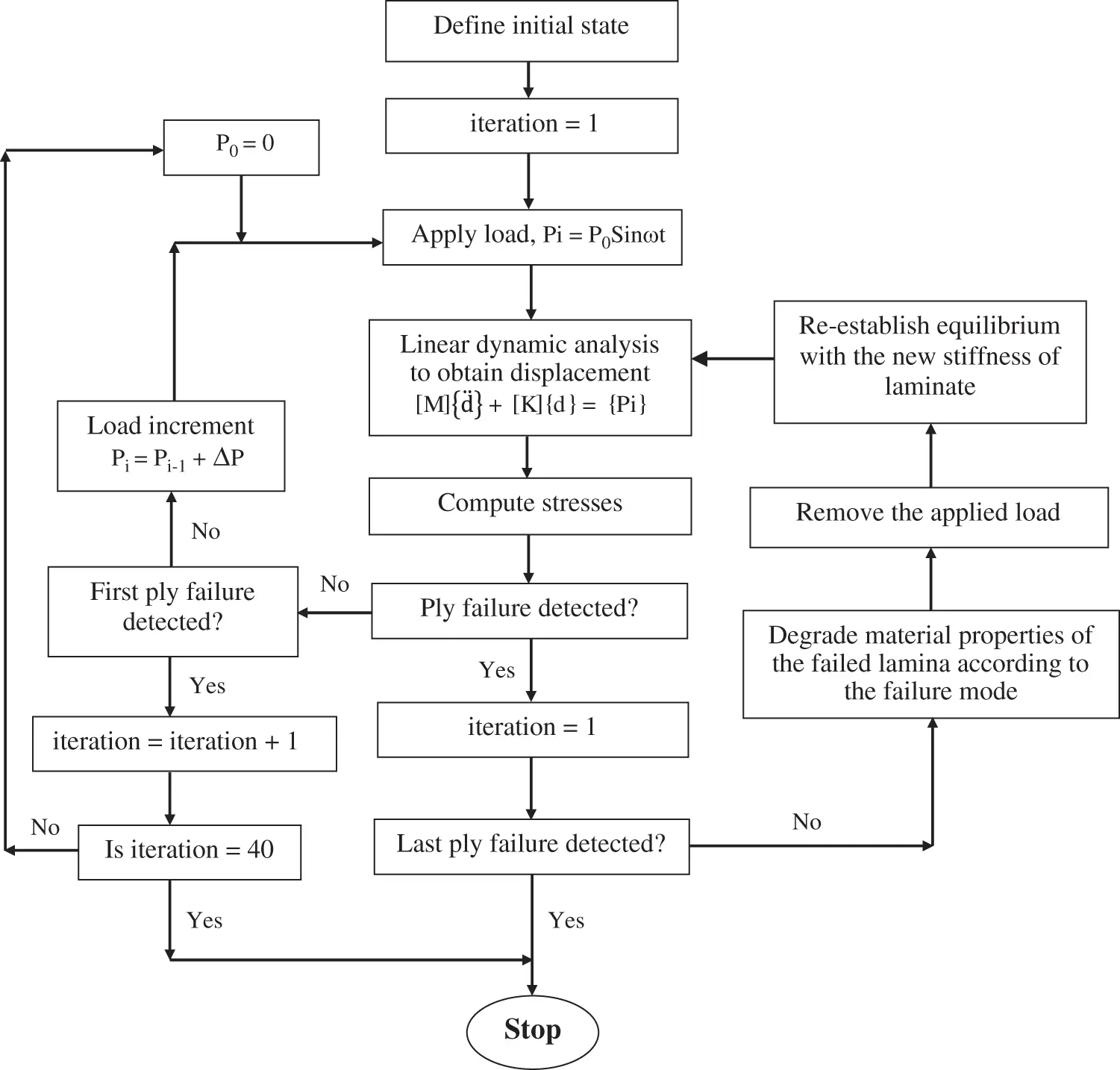Figure 1: Flowchart of the failure analysisSymmetric and anti-symmetric four-ply laminates made up of T300/5208 graphite-epoxy composite material are investigated in the present study. The laminate dimensions are 228.6 mm in length, 127.0 mm in width, and 0.127 mm in thickness. The mass density of the laminates investigated is 1.5757 × 10-6 kg/mm3. The strength parameters and material properties are provided in Tab. 2. Laminates with the same material and geometrical properties were also investigated by Reddy et al. , Pal & Ray , Pal & Bhattacharyya  and Pal & Bhar  for the first ply failure analysis. The lamination schemes used for the investigation are given in Tab. 3. Where θ is the fibre orientation angle in radian and for laminate types C and D, θ is varied from 0° to 90° at an interval of 15°.

Table 2: Material properties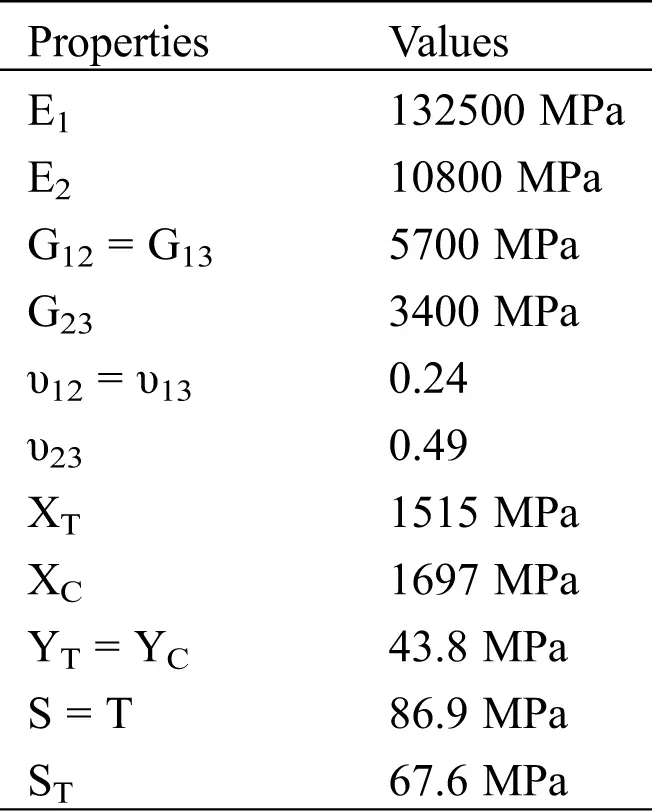Table 3: Lamination schemes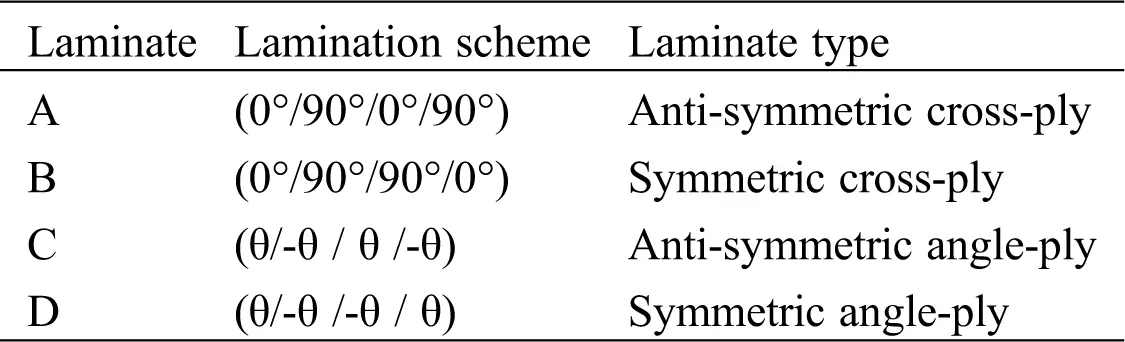A convergence study is carried out to determine the appropriate mesh size required for the analysis to achieve a satisfactory result. Fig. 2 shows the convergence study for simply supported anti-symmetric and symmetric cross-ply laminates under a sinusoidal dynamic load at the fundamental frequency and an amplitude of 0.001 MPa. The results of central deflection are recorded after the first iteration of the Newmark-beta method. The convergence is achieved at 8 × 8 mesh division. For the validation of the coded methodology, first ply failure load of a four-ply symmetric cross-ply laminate with all edges simply supported (type-B) under uniform transverse static pressure is determined for different failure theories. The results obtained are presented in Tab. 4 and were found to be in good agreement with those reported by Pal & Bhattacharya .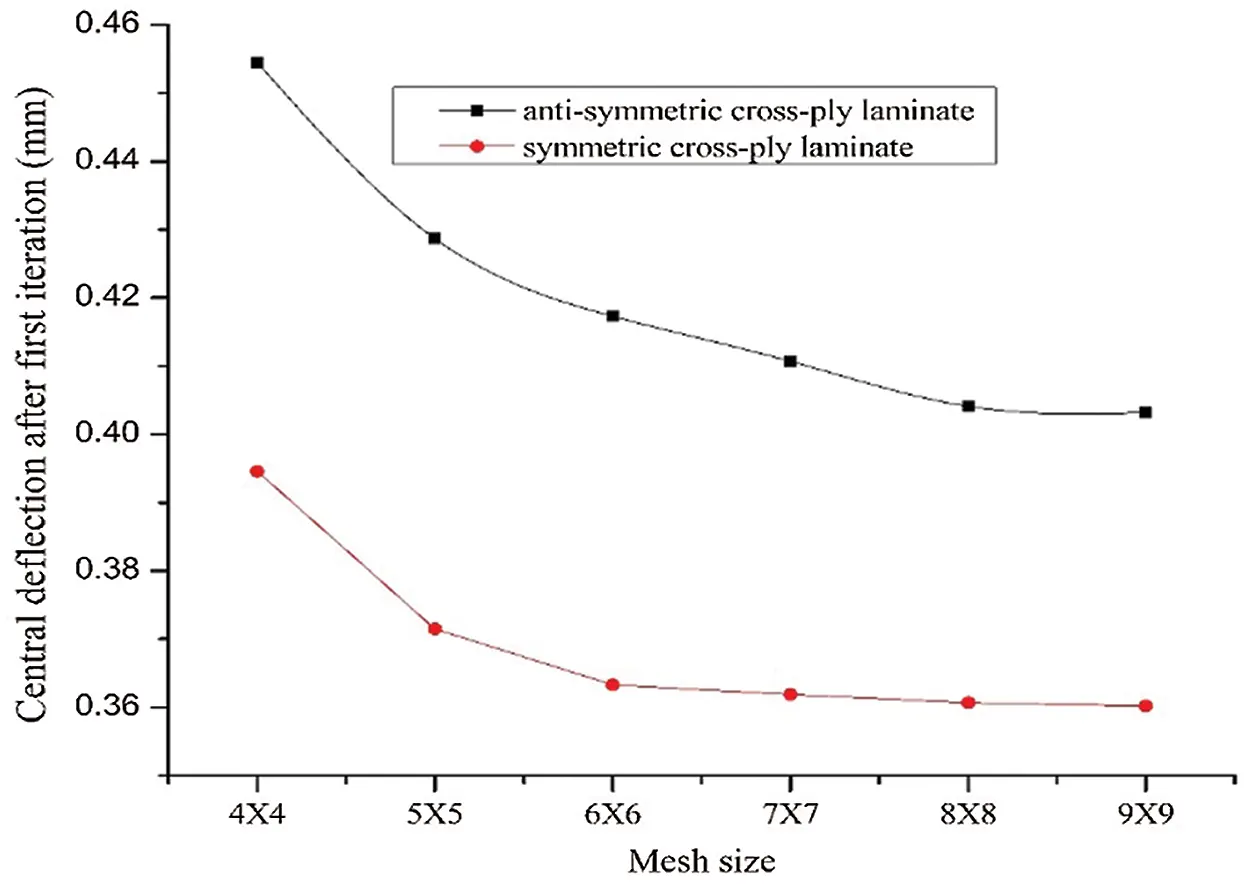Figure 2: Convergence study for anti-symmetric and symmetric cross-ply laminates

Table 4: Validation of results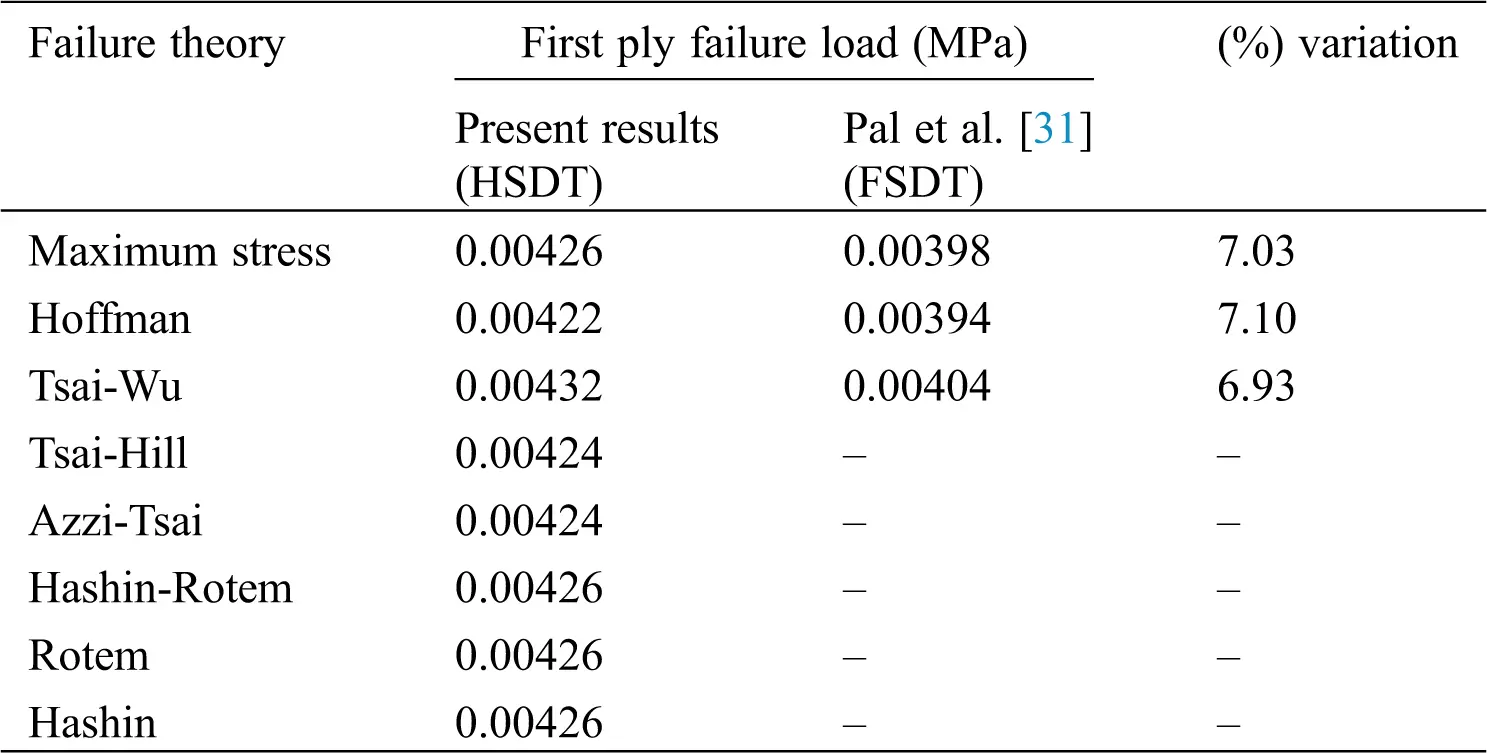The coded methodology provides acceptable results for different failure theories, as shown in Tab. 4. The dynamic failure loads are then determined from the developed finite element code for symmetric and anti-symmetric laminates for the lamination schemes listed in Tab. 3.

5.1 5.1 Dynamic Failure Load of Simply Supported Laminates

The structure's dynamic response depends on the amplitude and frequency of applied load, mass and stiffness of the structure, and fundamental frequency of the structure. The fundamental frequencies of simply supported laminates are evaluated from Eq. (35) using the Jacobi iterative technique and are presented in Tab. 5. Typical time response of central deflection of both the anti-symmetric and symmetric cross-ply laminates under sinusoidal forcing amplitude of 0.001 MPa at the fundamental frequency is shown in Fig. 3. It is found that the deflection increases with time. For a given amplitude, the dynamic response depends only on the frequency ratio since the structure's mass and stiffness are constant. The maximum effect of a dynamic load occurs when the frequency ratio is one, i.e., when the load is applied at the fundamental frequency or resonant frequency.

Table 5: Fundamental frequency (radian/sec) of simply supported laminates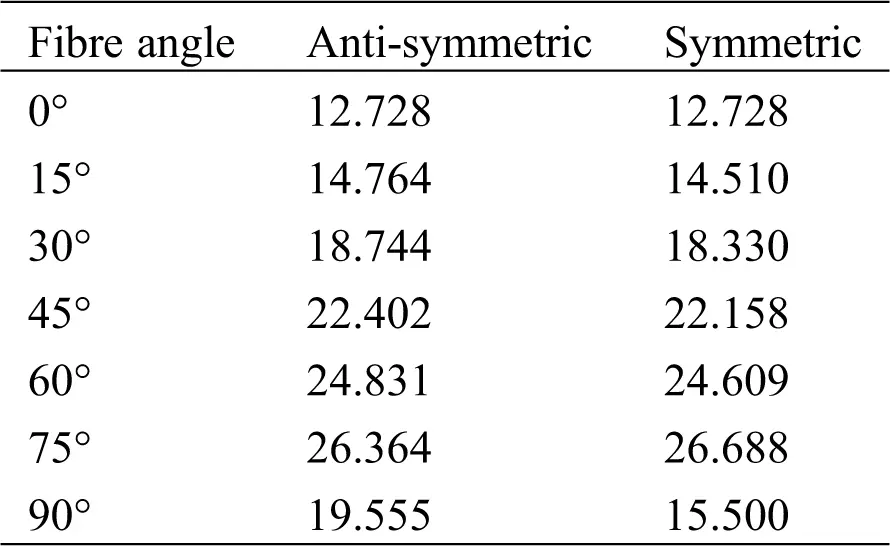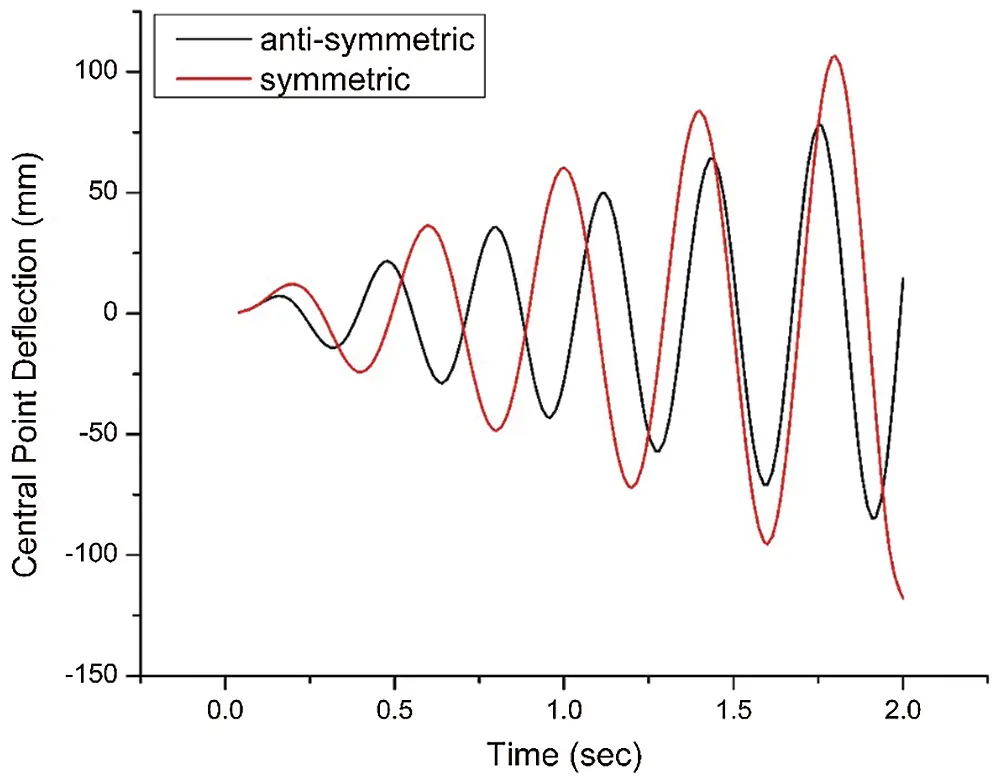Figure 3: Central deflection of anti-symmetric and symmetric cross-ply laminates

In this study, the failure loads are determined under the sinusoidal dynamic load of P0sinωt, where P0 implies the amplitude of load, and ω is the frequency of the load. A time step of 0.04 sec is taken for determining the dynamic response of each laminate by using the Newmark-beta method. Therefore, ten numbers of iteration imply a dynamic load for a duration of 0.4 sec. Initially, a load of very small amplitude is applied and checked for the failure. If no failure is detected during the load duration of 0.4 sec, the amplitude is increased by a small amount and the process is repeated. If a failure is detected, the load is immediately removed and the structure is allowed to vibrate freely. The failure may progress after first ply to some successive plies during the free vibration. The ply failure is designated by M (matrix failure) or F (fibre failure) depending upon whether the matrix or the fibre has failed. M and F are preceded by failed ply-number with top most ply numbered as 1 and bottom most ply as 4 for the four-ply laminates investigated. The computed failure loads of symmetric and anti-symmetric simply supported laminates are presented in Tab. 6. The failure progression of laminates is presented in Tab. 7.

Table 6: First ply failure load (MPa×10-3) of anti-symmetric (B & C) and symmetric (A & D) laminates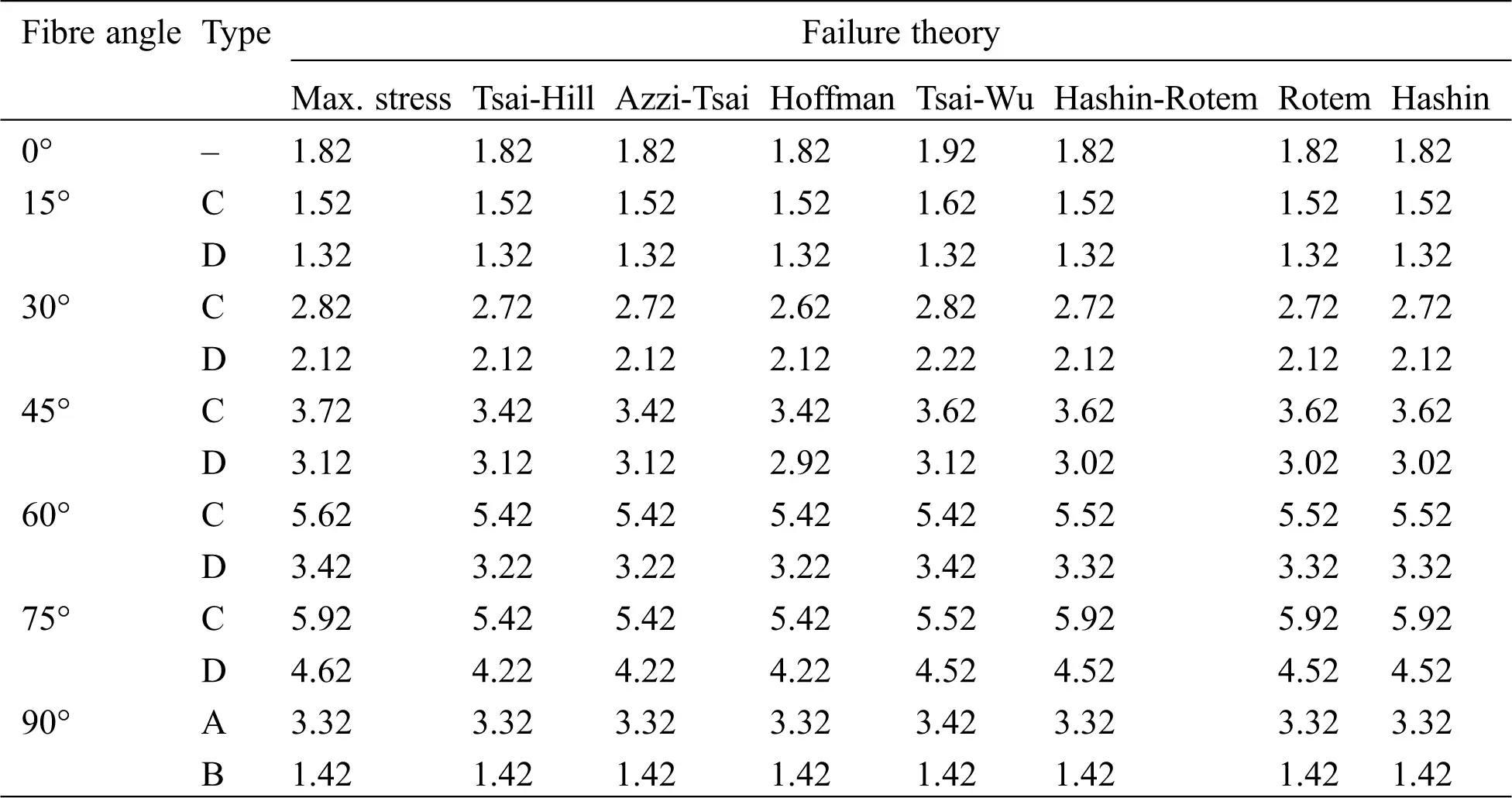Table 7: Failure progression of anti-symmetric (B & C) and symmetric (A & D) laminates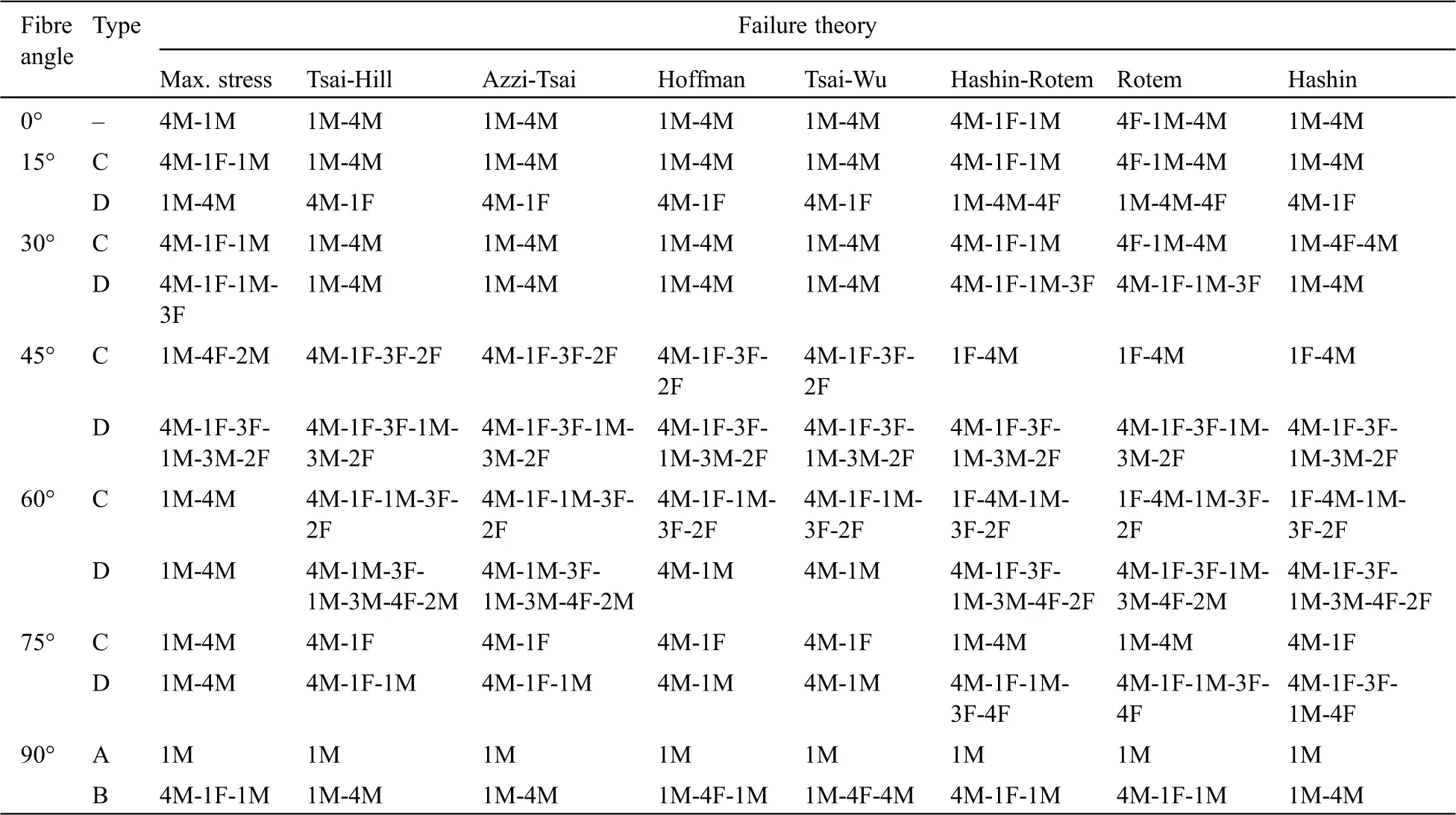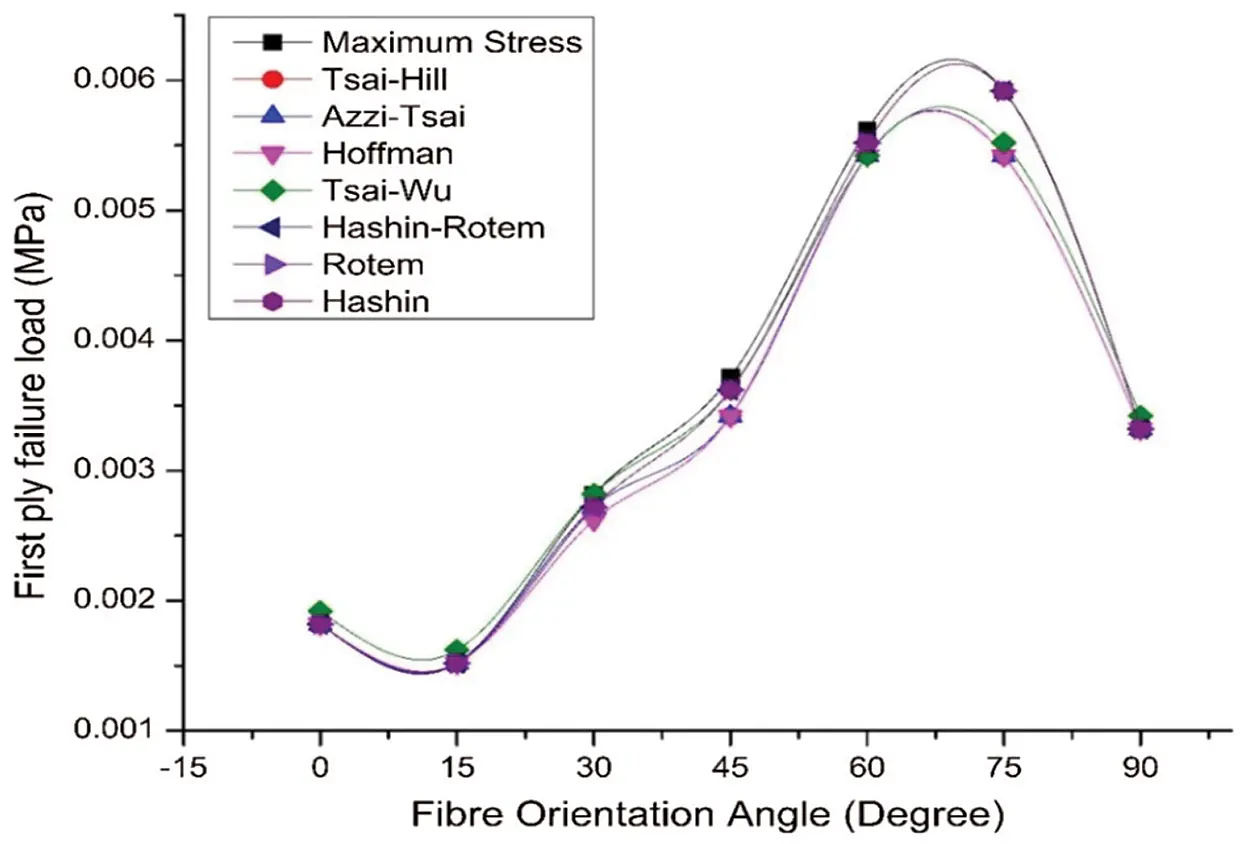Figure 4: First ply failure load vs fibre orientation of anti-symmetric laminate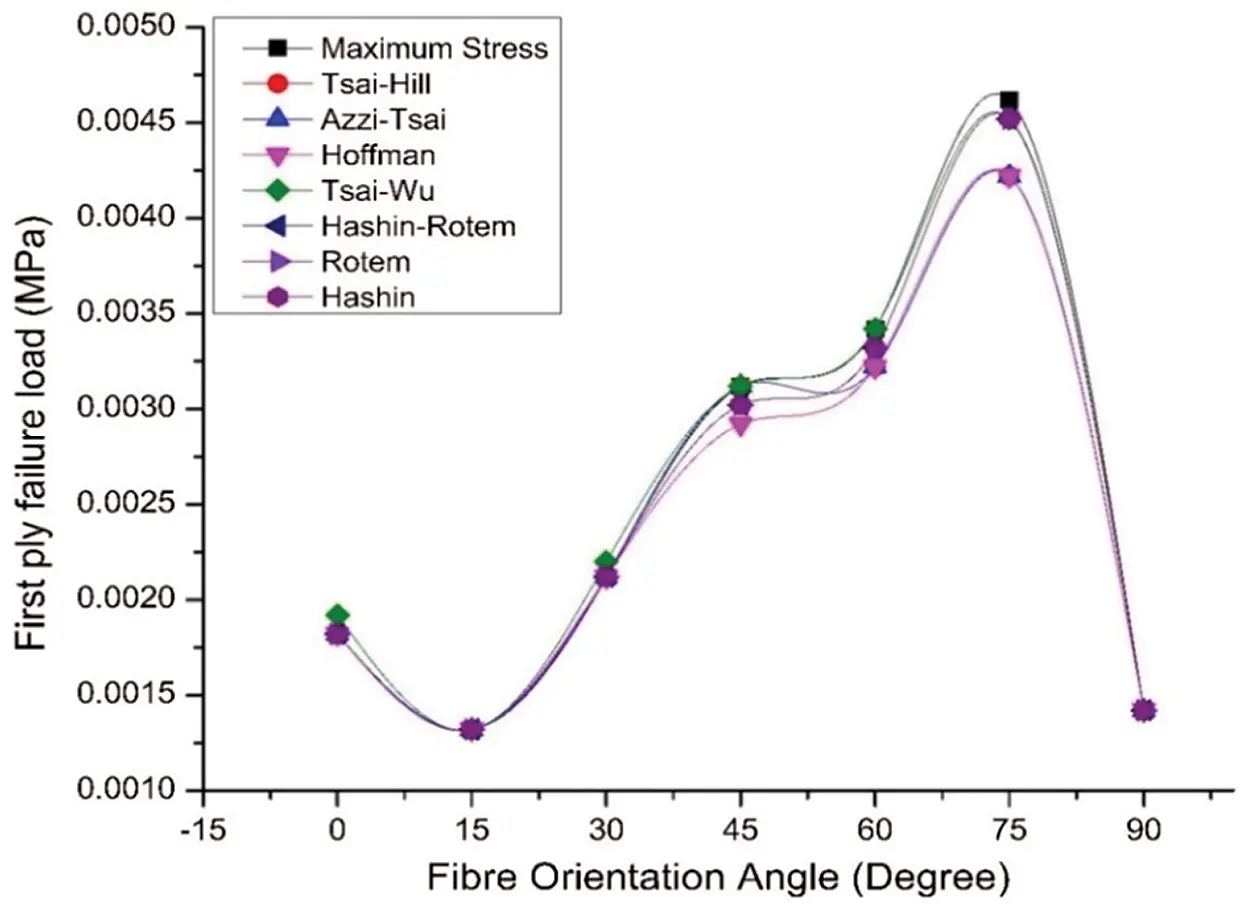Figure 5: First ply failure load vs fibre orientation of symmetric laminate

5.2 Dynamic Failure Load of Clamped Laminates

Likewise, the fundamental frequencies of clamped laminates are presented in Tab. 8. The central deflection of anti-symmetric and symmetric cross-ply laminates under sinusoidal forcing amplitude of 0.001 MPa at the fundamental frequency is demonstrated in Fig. 6. A similar phenomenon is observed as the applied frequency is close to the fundamental frequency. The failure loads are determined at the resonant frequency for a duration of 0.4 s. The computed failure loads are presented in Tab. 9 for clamped laminates. The failure progression of laminates is presented in Tab. 10. The failure load of clamped laminates is normally higher than simply supported laminates. The failure load of anti-symmetric laminates is generally higher than their respective symmetric laminates. The failure progresses to a greater number of ply failures as the fibre orientation increases for both the anti-symmetric and symmetric angle-ply laminates. As compared to simply supported laminate’s failure, the clamped laminates have generally progressed to a greater number of ply failures. The first ply failure loads of anti-symmetric and symmetric clamped laminates are illustrated in Figs. 7 and 8, respectively. The first ply failure load for anti-symmetric as well as symmetric laminate constantly increases from 0° to 75° then decreases till 90° for all the cases of failure theories. The failure loads for all the failure theories are the same for most of the cases even though the failed ply and failure mode may be different. The failure loads show that all the failure theories are almost equally capable of predicting the first ply failure.

Table 8: Fundamental frequency (radian/sec) of clamped laminates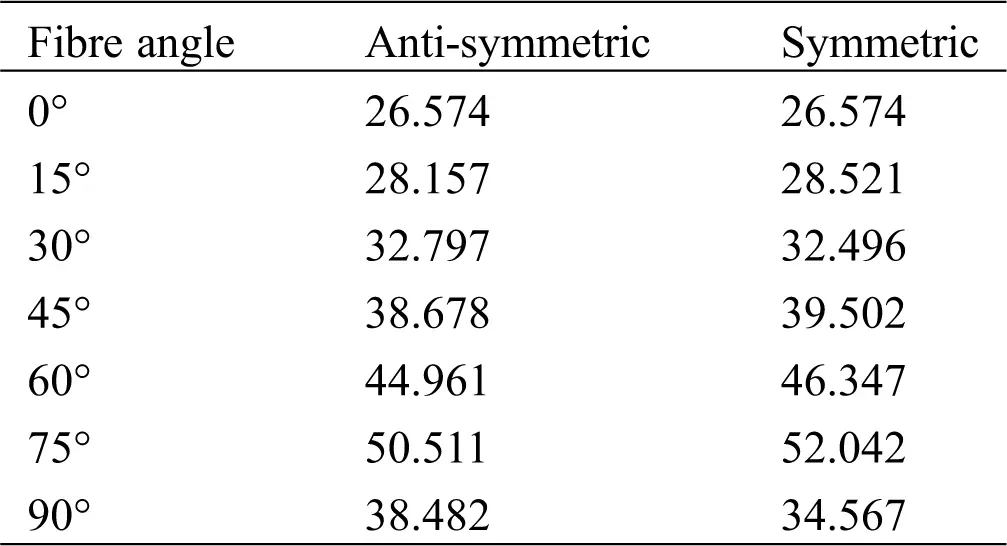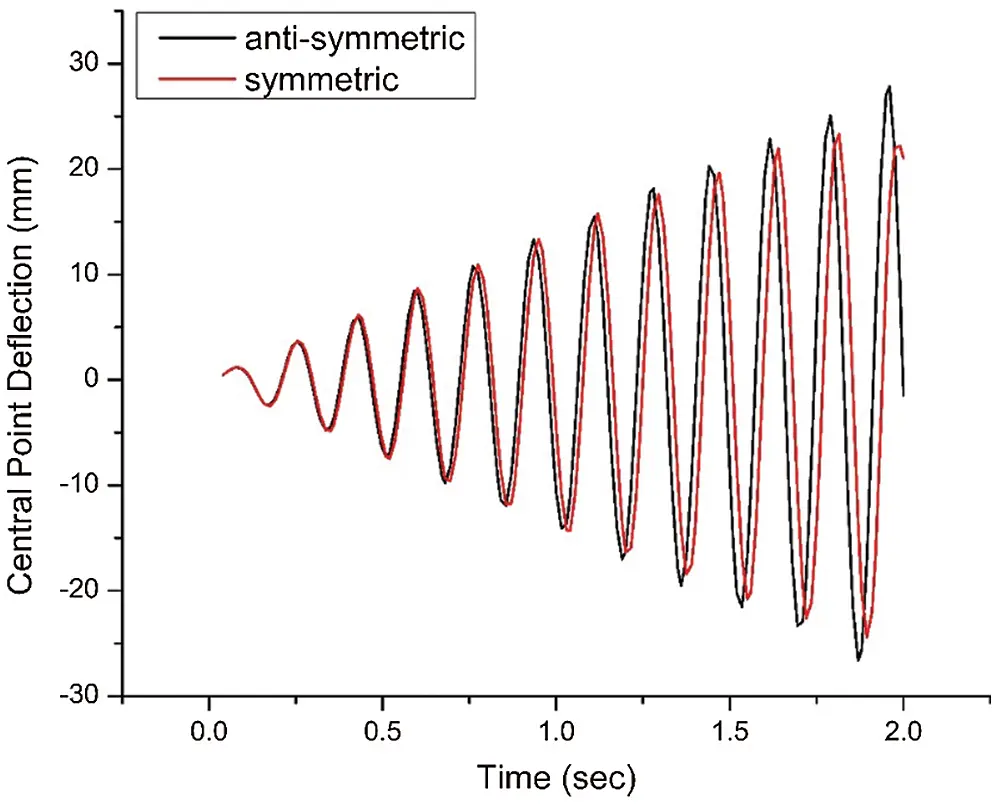Figure 6: Central deflection of anti-symmetric and symmetric cross-ply laminates

Table 9: First Ply failure load (MPa×10-3) of anti-symmetric (B & C) and symmetric (A & D) laminatesTable 10: Failure progression of anti-symmetric (B & C) and symmetric (A & D) laminates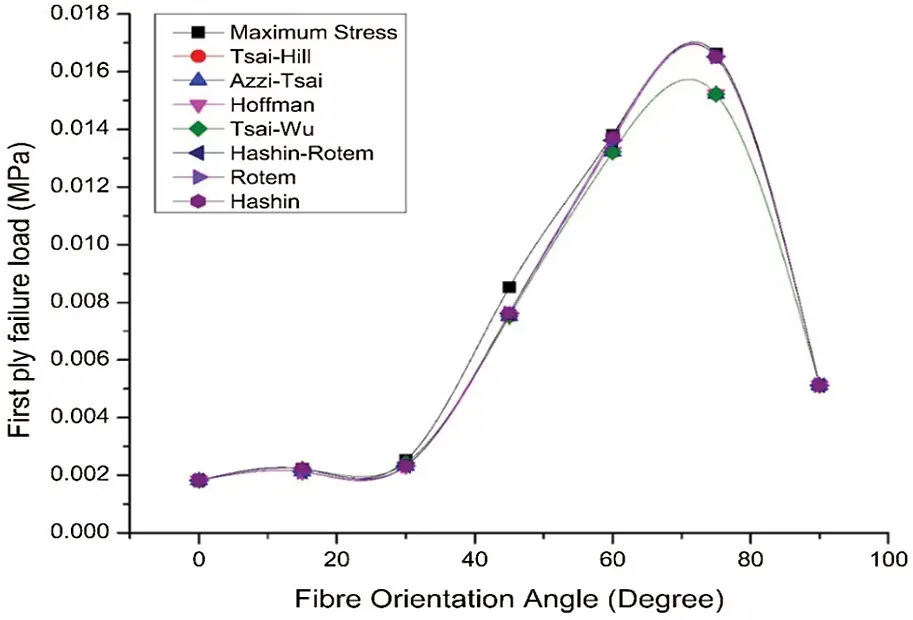Figure 7: First ply failure load vs fibre orientation of anti-symmetric laminate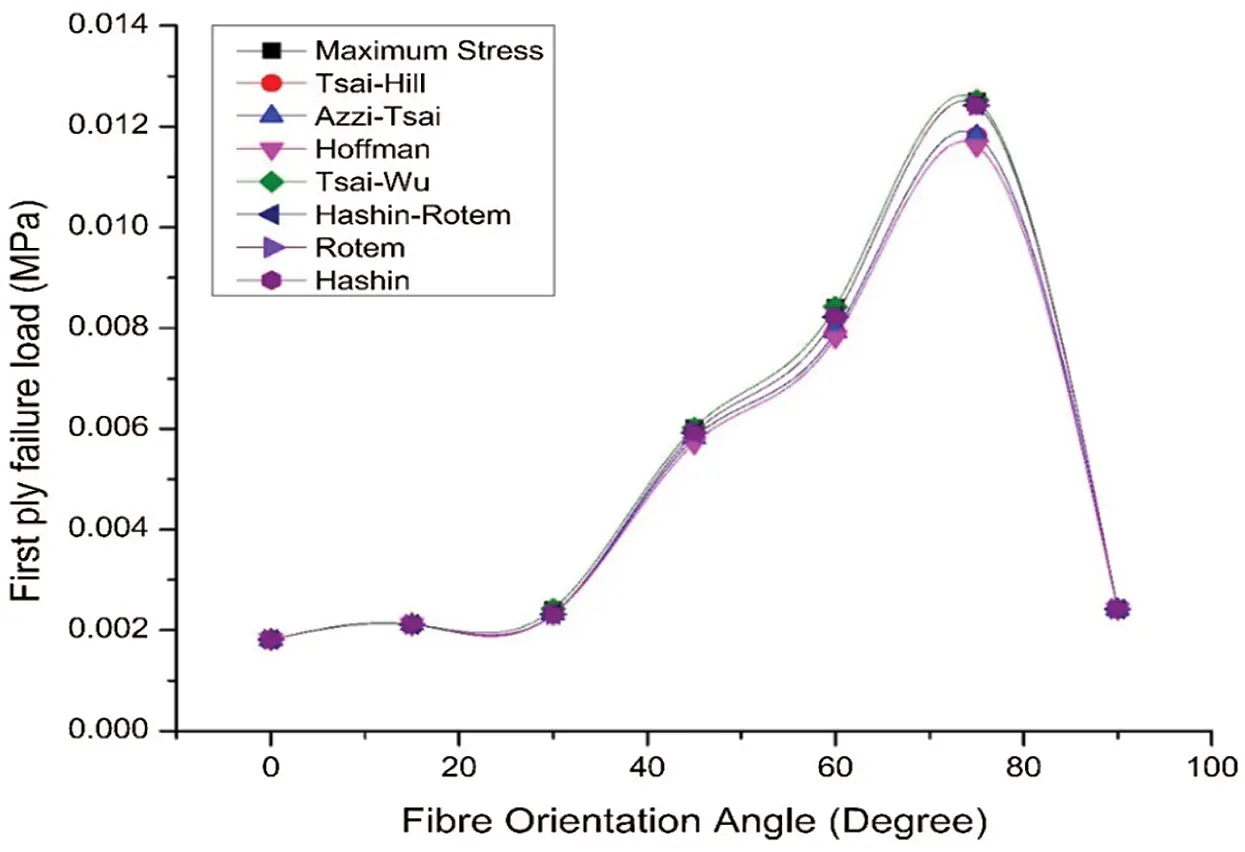Figure 8: First ply failure load vs fibre orientation of symmetric laminate

6  Conclusions

The behaviour of laminates under uniform transverse sinusoidal dynamic pressure was investigated during its successive failure. The prediction of failure load, mode of failure, failed lamina and progression of failure were studied by different failure theories. The following conclusions are drawn based on the investigation carried out:

•   The first ply failure loads predicted by all the theories are close to each other and even the same for most cases, which shows that they are almost equally capable of predicting the first ply failure load.

•   A dynamic load at the fundamental frequency of a laminate can cause the first ply failure and lead to successive ply failures even after the load is removed.

•   For simply supported angle-ply laminates, the failure load increases with fibre orientation after 15° fibre orientation, whereas for clamped laminates, it increases with fibre orientation for all the cases.

•   The anti-symmetric laminates are generally stronger (greater first ply failure load) than their respective symmetric laminates for both simply supported and clamped edges. The symmetric laminates are more susceptible to dynamic loads as compared to anti-symmetric laminates.

•   The progression of failure after removing the load as predicted by different failure theories is generally not the same. The mode determining failure theories predict the failure to a greater extent than other failure theories for most of the simply supported laminates.

•   Failure progresses to a greater number of ply failures as the fibre orientation increases for both simply supported and clamped laminates. Failure in clamped laminates is generally progressed to a greater number of ply failures than simply supported laminates.

Funding Statement: The author(s) received no specific funding for this study.

Conflicts of Interest: The authors declare that they have no conflicts of interest to report regarding the present study.

## References

1. Srinivas, S., Rao, C. V. J., & Rao, A. K. (1970). An exact analysis for vibration of simply-supported homogeneous and laminated thick rectangular plates. Journal of Sound and Vibration, 12(2), 187-199. [Google Scholar] [CrossRef]
2. Ye, J. Q. (1997). A three-dimensional free vibration analysis of cross-ply laminated rectangular plates with clamped edges. Computer Methods in Applied Mechanics and Engineering, 140(3-4), 383-392. [Google Scholar] [CrossRef]
3. Shi, J. W., Nakatani, A., & Kitagawa, H. (2004). Vibration analysis of fully clamped arbitrarily laminated plate. Composite Structures, 63(1), 115-122. [Google Scholar] [CrossRef]
4. Zhen, W., & Wanji, C. (2006). Free vibration of laminated composite and sandwich plates using global-local higher-order theory. Journal of Sound and Vibration, 298(1-2), 333-349. [Google Scholar] [CrossRef]
5. Qatu, M. S. (1991). Free vibration of laminated composite rectangular plates. International Journal of Solids and Structures, 28(8), 941-954. [Google Scholar] [CrossRef]
6. Khdeir, A. A., & Reddy, J. N. (1999). Free vibrations of laminated composite plates using second-order shear deformation theory. Computers & Structures, 71(6), 617-626. [Google Scholar] [CrossRef]
7. Zhang, Y. H., & Yang, C. H. (2009). Recent developments in finite element analysis for laminated composite plates. Composite Structures, 88(1), 147-157. [Google Scholar] [CrossRef]
8. Thai, H. T., & Kim, S. E. (2010). Free vibration of laminated composite plates using two variable refined plate theory. International Journal of Mechanical Sciences, 52(4), 626-633. [Google Scholar] [CrossRef]
9. Mahi, A., Bedia, E. A. A., & Tounsi, A. (2015). A new hyperbolic shear deformation theory for bending and free vibration analysis of isotropic, functionally graded, sandwich and laminated composite plates. Applied Mathematical Modelling, 39(9), 2489-2508. [Google Scholar] [CrossRef]
10. Kalita, K., Ramachandran, M., Raichurkar, P., Mokal, S. D., & Haldar, S. (2016). Free vibration analysis of laminated composites by a nine node isoparametric plate bending element. Advanced Composites Letters, 25(5), 108-116. [Google Scholar] [CrossRef]
11. Zhang, L. W., & Selim, B. A. (2017). Vibration analysis of CNT-reinforced thick laminated composite plates based on Reddy’s higher-order shear deformation theory. Composite Structures, 160, 689-705. [Google Scholar] [CrossRef]
12. Singh, D. B., & Singh, B. N. (2017). New higher order shear deformation theories for free vibration and buckling analysis of laminated and braided composite plates. International Journal of Mechanical Sciences, 131–132(2), 265-277. [Google Scholar] [CrossRef]
13. Houmat, A. (2018). Three-dimensional free vibration analysis of variable stiffness laminated composite rectangular plates. Composite Structures, 194, 398-412. [Google Scholar] [CrossRef]
14. Shia, P., Dong, C., Sun, F., Liu, W., & Hu, Q. (2018). A new higher order shear deformation theory for static vibration and buckling responses of laminated plates with the isogeometric analysis. Composite Structures, 204(1), 342-358. [Google Scholar] [CrossRef]
15. Khdeir, A. A., & Reddy, J. N. (1988). Dynamic response of antisymmetric angle-ply laminated plates subjected to arbitrary loading. Journal of Sound and Vibration, 126(3), 437-445. [Google Scholar] [CrossRef]
16. Khdeir, A. A. (1995). Forced vibration analysis of antisymmetric angle-ply laminated plates with various boundary conditions. Journal of Sound and Vibration, 188(2), 257-267. [Google Scholar] [CrossRef]
17. Alexander, B., & Friedrich, K. (1994). Initial and progressive failure analysis of laminated composite structures under dynamic loading. Composite Structures, 27(4), 439-456. [Google Scholar] [CrossRef]
18. Chen, J., & Dawe, D. J. (1996). Linear transient analysis of rectangular laminated plates by a finite strip-mode superposition method. Composite Structures, 35(2), 213-228. [Google Scholar] [CrossRef]
19. Swaddinudhipong, S., & Liu, Z. S. (1997). Response of laminated composite plates and shells. Composite Structures, 37(1), 21-32. [Google Scholar] [CrossRef]
20. Jam, J. E., & Nia, N. G. (2007). Dynamic failure analysis of laminated composite plates. Metalurgija, 13(3), 187-196. [Google Scholar]
21. Bastürk, S., Uyanık, H., & Kazanci, Z. (2014). An analytical model for predicting the deflection of laminated basalt composite plates under dynamic loads. Composite Structures, 116(9), 273-285. [Google Scholar] [CrossRef]
22. Ray, C., & Majumder, S. (2014). Failure analysis of composite plates under static and dynamic loading. Structural Engineering and Mechanics, 52(1), 137-147. [Google Scholar] [CrossRef]
23. Liu, P. F., Liao, B. B., & Jia, L. Y. (2017). Finite element analysis of dynamic progressive failure of plastic composite laminates under low velocity impact. Composite Structures, 159, 567-578. [Google Scholar] [CrossRef]
24. Soufeiani, L., Ghadyani, G., Kueh, A. B. H., & Nguyen, K. T. Q. (2017). The effect of laminate stacking sequence and fiber orientation on the dynamic response of FRP composite slabs. Journal of Building Engineering, 13, 41-52. [Google Scholar] [CrossRef]
25. Adhikari, B., & Singh, B. N. (2018). An efficient higher order non-polynomial Quasi 3-D theory for dynamic responses of laminated composite plates. Composite Structures, 189(1), 386-397. [Google Scholar] [CrossRef]
26. Joshi, R., Pal, P., & Duggal, S. K. (2020). Ply-by-ply failure analysis of laminates using finite element method. European Journal of Mechanics - A/Solids, 81(6), 103964. [Google Scholar] [CrossRef]
27. Joshi, R., & Duggal, S. K. (2020). Free vibration analysis of laminated composite plates during progressive failure. European Journal of Mechanics - A/Solids, 83(–132), 104041. [Google Scholar] [CrossRef]
28. Tolson, S., & Zabaras, N. (1991). Finite element analysis of progressive failure in laminated composite plates. Computers & Structures, 38(3), 361-376. [Google Scholar] [CrossRef]
29. Reddy, J. N., & Pandey, A. K. (1987). A first-ply failure analysis of composite laminates. Computers & Structures, 25(3), 371-393. [Google Scholar] [CrossRef]
30. Pal, P., & Ray, C. (2002). Progressive failure analysis of laminated composite plates by finite element method. Journal of Reinforced Plastics and Composites, 21(16), 1505-1513. [Google Scholar] [CrossRef]
31. Pal, P., & Bhattacharya, S. K. (2007). Progressive failure analysis of cross-ply laminated composite plates by finite element method. Journal of Reinforced Plastics and Composites, 26(5), 65-77. [Google Scholar]
32. Pal, P., & Bhar, A. (2013). The displacement perspective during ultimate failure of composite laminates. Applied Composite Materials, 20(2), 171-183. [Google Scholar] [CrossRef]This work is licensed under a Creative Commons Attribution 4.0 International License, which permits unrestricted use, distribution, and reproduction in any medium, provided the original work is properly cited.